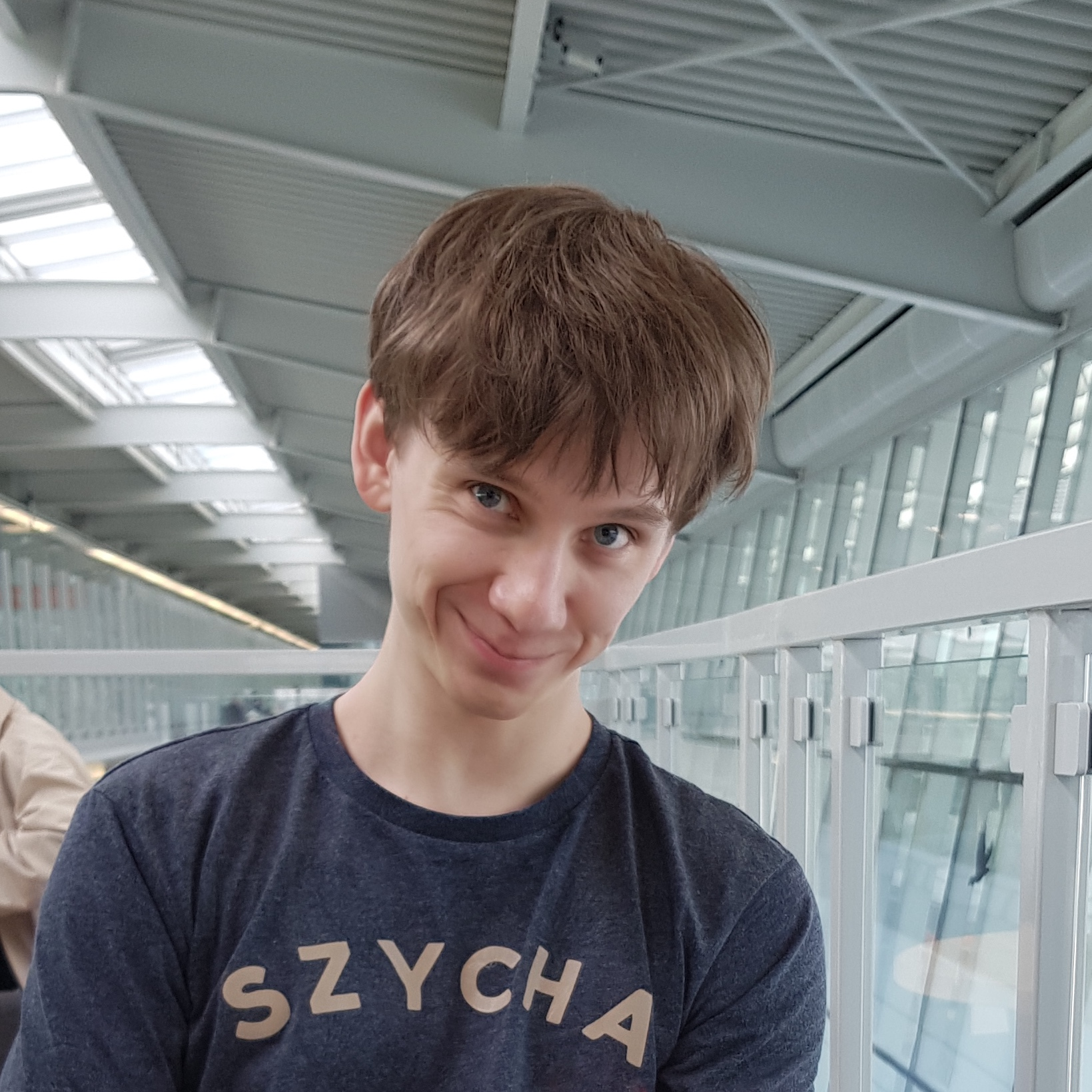### Tomek Korbak

PhD student,
University of Sussex

# Training a compute-optimal gpt2-small

Assume you’d like to train a gpt2-small-sized model (117m parameters). What is the optimal training set size? I’ll try to estimate that number following Training Compute-Optimal Large Language Models (also known as “the Chinchilla paper”).

## Background: Chinchilla scaling law

The Chinchilla paper presents a scaling law for language modelling loss $$L$$ as a function of model size $$N$$ (the number of parameters) and training dataset size $$D$$ (the number of tokens). According to their model, language model’s loss is a sum of thee terms:

$L(N,D) = \frac{A}{N^\alpha} + \frac{B}{D^\beta} + E.$

Each term has an intuitive meaning: $$E$$ is a constant roughly equal the entropy of natural language (or, whatever your training distribution is). An infinitely big model trained on infinitely many tokens would approach $$E$$. The first and second terms are penalties paid for, respectively, having a finite model and a finite dataset. (A discussion can be found here.)

The Chinchilla paper paper estimates $$A = 406.4$$, $$B = 410.7$$, $$E = 1.69$$, $$\alpha = 0.32$$ and $$\beta = 0.28$$by fitting a regression model to a dataset of 400 language model training runs. Given these parameters, one can predict, for example, that a loss obtained by training a 280B parameter language model on 300B tokens of data (this corresponds to Gopher) results in loss $$L(280 \cdot 10^9, 300 \cdot 10^9) = 1.993$$.

What’s more interesting, one can estimate an optimal allocation of a fixed compute budget $$C$$. Training a model with $$N$$ parameters od $$D$$ tokens incurs a cost of $$C = 6ND$$ floating-point operations (FLOPs) (see Appendix F). A compute-optimal model for a fixed $$C$$ is a combination of $$N$$ and $$D$$ satisfying the $$C = 6ND$$ constraint such that the loss $$L(N, D)$$ is minimal. In other words, either increasing model size (at an expense of dataset size) or dataset size (at an expense of model size) results in higher loss. Such $$N$$ and $$D$$ can be found in closed form, see eq. 4 in the paper.

## Chinchilla model predictions for gpt2-small

The three plots below shows predictions of the Chinchilla model for $$N_\text{gpt2-sm} = 117 \cdot 10^6$$. The firsts two plots show loss as a function of $$D$$ and $$C = 6N_\text{gpt2-sm}D$$. Intuitively, they show the shape of train loss predicted by the Chinchilla model. The last plot gives a very rough estimate of a price of a training run assuming a 1.2e17 FLOP/dollar from Ajeya Cotra’s report (Appendix).## Compute-optimal dataset size

The Chinchilla paper focuses on compute-optimal models: optimal $$(N, D)$$ for a fixed $$D$$. I’m interested in an inverse problem: what’s an optimal dataset size $$D$$ for a model size $$N$$. Equivalently, what’s an optimal compute budget $$C$$ for a model of size $$N$$. There are two intuitive framings of this question:

1. When should I stop training? What’s the number of tokens $$D$$ such that after $$D$$ subsequent decreases in my loss ($$L(N, D+1), L(N, D+2), \dots)$$ are small enough that I’d be better off spending my $$6N(D+1)$$ FLOPs training a bigger model on fewer tokens.
2. How long should I keep training? What’s the number of tokens $$D$$ that I need to reach to justify training a model with as many as $$N$$ parameters (as opposed to a training a smaller model on more tokens)?

Therefore, a dataset size $$D$$ is compute-optimal for model size $$N$$ if $$(N, D)$$ is compute-optimal: every other allocation of $$6ND$$ FLOPs results in a worse loss:

$L(N-1, \frac{N}{N-1}D) > L(N, D) < L(N+1, \frac{N}{N+1}D).$

## Compute-optimal dataset for gpt2-small

First, we can plot IsoFLOP curves for a range of compute budgets $$10^{16} < C < 10^{20}$$. An IsoFLOP curve for budget $$C$$ shows loss as a function of $$N$$, i.e. $$L(N, \frac{C}{6N})$$.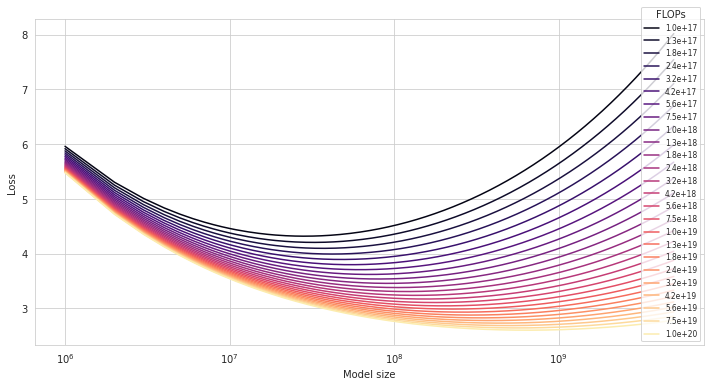Second, we can find a minimum of each IsoFLOP with respect to $$N$$. Each such minimum (marked by a green dot) is a compute-optimal model $$(N, D)$$ for a given budget $$C$$.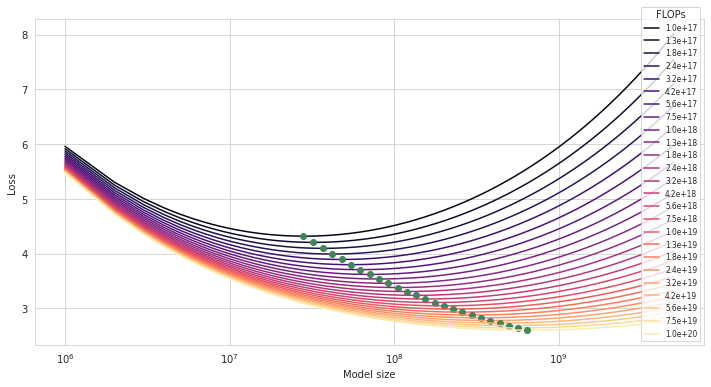Finally, we can look for a compute-optimal model $$(N, D)$$ with $$N$$ closest to $$N_\text{gpt2-sm} = 117 \cdot 10^6$$.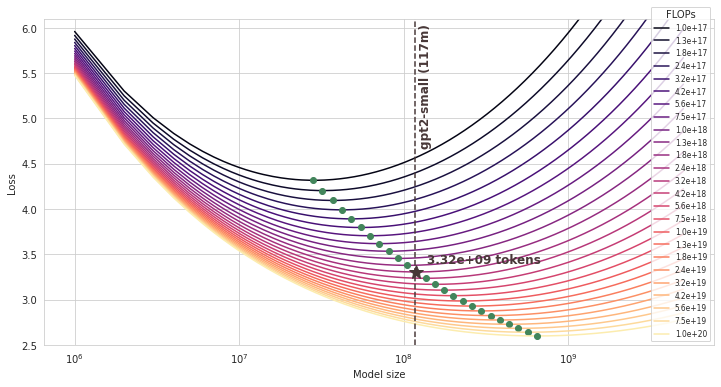The compute-optimal dataset size turns out to be 3.32B tokens (and the corresponding compute budget is 2.37E+18 FLOPs).

This seems to match results from the Chinchilla paper: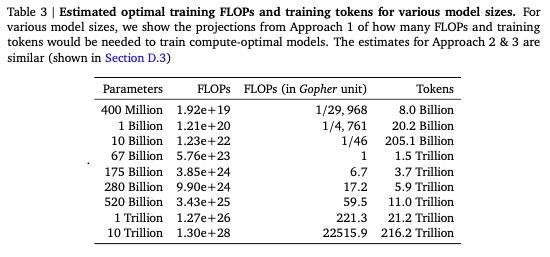## Postscriptum

### How much does it cost?

Less than $100 on the cloud. Based on my recent experiments, with two A100 (80gb) GPUs, it takes around 16h to train gpt2-small on 3.3B tokens. You’d pay$80 for 4xA100 (40gb) on Lambda Labs or around \$45 on GCP, in a good region.

### How many parameters does gpt2-small have?

While the GPT2 paper says gpt2-small has 117m parameters, the Hugginface implementation actually has 127m:

from transformers import AutoModel
gpt2_small = AutoModel.from_pretrained('gpt2')
gpt2_small.num_parameters()


Not counting embeddings (gpt2_small.num_parameters(exclude_embeddings=True)), it’s 85m which is also quite off. I’m not sure what’s going on here. The difference doesn’t seem to matter much.

### What are good hyperparameters?

I found it useful to look at config files of two well-documented open source projects training gpt2-small-sized models: codeparrot and mistral. A Bloom paper called What Language Model to Train if You Have One Million GPU Hours? and the Gopher paper also report some results with gpt2-small-sized models.

Overall, I’d use a linear or cosine learning rate schedule (with warmup_ratio=0.01) and do a sweep over batch sizes and learning rates. For instance, codeparrot-small used learning rate 5e-4 and batch size 192 (197k tokens) while mistral used learning rate 6e-4 and batch size 512 (524k tokens). Similarly, the Gopher paper reports their learning rate and batch size for 117m model to be 6e-4 and 125 (0.25m tokens; note they have context window 2048).